# HSSlive: Plus One & Plus Two Notes & Solutions for Kerala State Board

## BSEB Class 10 Maths Chapter 6 Triangles Ex 6.4 Textbook Solutions PDF: Download Bihar Board STD 10th Maths Chapter 6 Triangles Ex 6.4 Book AnswersBSEB Class 10 Maths Chapter 6 Triangles Ex 6.4 Textbook Solutions PDF: Download Bihar Board STD 10th Maths Chapter 6 Triangles Ex 6.4 Book Answers

BSEB Class 10th Maths Chapter 6 Triangles Ex 6.4 Textbooks Solutions and answers for students are now available in pdf format. Bihar Board Class 10th Maths Chapter 6 Triangles Ex 6.4 Book answers and solutions are one of the most important study materials for any student. The Bihar Board Class 10th Maths Chapter 6 Triangles Ex 6.4 books are published by the Bihar Board Publishers. These Bihar Board Class 10th Maths Chapter 6 Triangles Ex 6.4 textbooks are prepared by a group of expert faculty members. Students can download these BSEB STD 10th Maths Chapter 6 Triangles Ex 6.4 book solutions pdf online from this page.

## Bihar Board Class 10th Maths Chapter 6 Triangles Ex 6.4 Books Solutions

 Board BSEB Materials Textbook Solutions/Guide Format DOC/PDF Class 10th Subject Maths Chapter 6 Triangles Ex 6.4 Chapters All Provider Hsslive

## How to download Bihar Board Class 10th Maths Chapter 6 Triangles Ex 6.4 Textbook Solutions Answers PDF Online?

2. Click on the Bihar Board Class 10th Maths Chapter 6 Triangles Ex 6.4 Answers.
3. Look for your Bihar Board STD 10th Maths Chapter 6 Triangles Ex 6.4 Textbooks PDF.
4. Now download or read the Bihar Board Class 10th Maths Chapter 6 Triangles Ex 6.4 Textbook Solutions for PDF Free.

Find below the list of all BSEB Class 10th Maths Chapter 6 Triangles Ex 6.4 Textbook Solutions for PDF’s for you to download and prepare for the upcoming exams:

## BSEB Bihar Board Class 10th Maths Solutions Chapter 6 Triangles Ex 6.4

Question 1.
Let ∆ ABC ~ ∆ DEF and their areas be, respectively, 64 cm² and 121 cm². If EF = 15.4 cm, find BC.
Solution: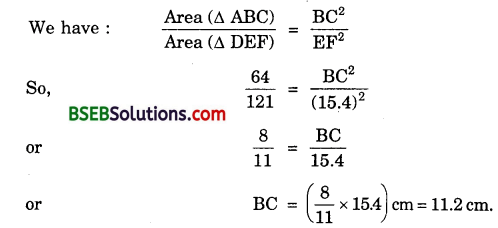Question 2.
Diagonals of a trapezium ABCD with AB || DC intersect each other at the point O. If AB = 2 CD, find the ratio of the areas of triangles AOB and COD.
Solution:
In ∆s AOB and COD, we have :
∠AOB = ∠COD [Vert.opp.∠s]
and ∠OAB = ∠OCD [Alternate ∠s]
∴ By AA criterion of similarity, we have: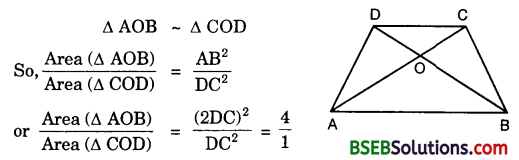Hence, area (∆ AOB) : area (∆ COD) = 4 : 1

Question 3.
In the figure, ABC and DBC are two triangles on the same base BC. If AD intersects BC at O, show that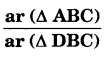= 𝐴𝑂𝐷𝑂.Solution:
Given : Two As ABC and DBC which stand on the same base BC but on the opposite sides of BC.
To prove :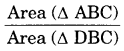= 𝐴𝑂𝐷𝑂.
Construction : Draw AE ⊥ BC and DF ⊥ BC.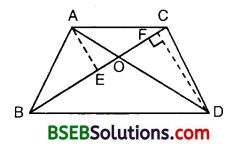Proof:
In ∆s AOE and DOF, we have :
∠AEO = ∠DFO = 90°
∠AOE = ∠DOF [Vertically opp. ∠s]
∴ By AA criterion of similarity, we have :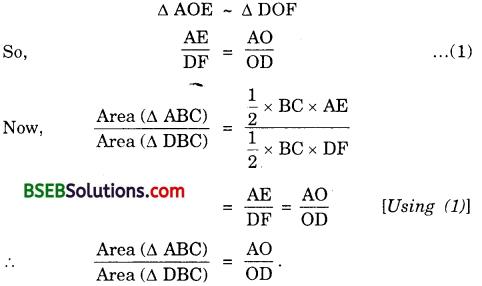Question 4.
If the areas of two similar triangles are equal, prove that they are congruent.
Solution:
Given : Two ∆s ABC and DEF such that ∆ ABC – ∆ DEF
and Area (∆ ABC) = Area (∆ DEF)
To prove : ∆ ABC ≅ ∆ DEF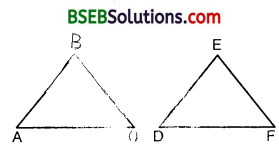Proof:
∆ ABC ~ ∆ DEF
So, ∠A = ∠D, ∠B = ∠E, ∠C = ∠F
and 𝐴𝐵𝐷𝐸 = 𝐵𝐶𝐸𝐹 = 𝐴𝐶𝐷𝐹
To establish ∆ ABC ≅ ∆ DEF, it is sufficient to prove that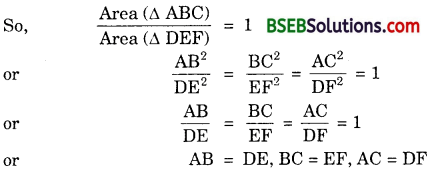Hence ∆ ABC ≅ ∆ DEF [By SSS]

Question 5.
D, E and F are respectively the mid-points of sides AB, BC and CA of ∆ ALBC. Find the ratio of the areas of ∆ DEF and ∆ ABC.
Solution:
Since D and E are respectively, the mid-points of the sides AB and BC of ∆ ABC, therefore
DE [| AC, or DE || FC … (1)
Since D and F are respectively the mid-points of the sides AB and AC
∆ ABC, thereforeDF || BC, or DF || EC … (2)
From (1) and (2), we can say that DECF is a parallelogram.
Now, in ∆s DEF and ABC, we have :
∠DEF = ∠A [Opp. Zs of ||gm ADEF]
and ∠EDF = ∠C [Opp. Zs of ||gm DECF]
∴ By AA criterion of similarity, we have :
∆ DE ≅ ∆ ACHence, Area (∆ DEF) : Area (∆ ABC) =1:4.

Question 6.
Prove that the ratio of the areas of two similar triangles is equal to the square of the ratio of their corresponding medians.
Solution:
Given : ∆ ABC ~ ∆ PQR, AD and PM are the medians of ∆s ABC and PQR respectively.
To Prove : Area (ΔABC) Area (ΔPQR)=AD2PM2
Proof : Since ∆ ABC ~ ∆ PQR, thereforeQuestion 7.
Prove that the area of an equilateral triangle described on one side of a square is equal to half the area of the equilateral triangle described on one of the diagonals.
Solution:
Given : A square ABCD. Equilateral ∆s BCE and ACF have been drawn on side BC and the diagonal AC respectively.
To prove : Area (∆ BCE) = 12(Area ∆ ACF)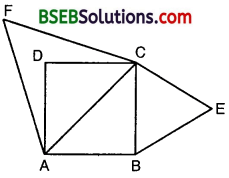Proof: ∆ BCE ~ ∆ ACF [All equilateral triangles are similar]Question 8.
ABC and BDE are two equilateral triangles such that D is the mid-point of BC. Ratio of the areas of triangles ABC and BDE is ____________.
(A) 2 : 1
(B) 1 : 2
(C) 4 : 1
(D) 1 : 4
Solution:
Since ∆ ABC and ∆ BDE are equilateral triangles, they are equiangular and hence ∆ ABC – ∆ BDE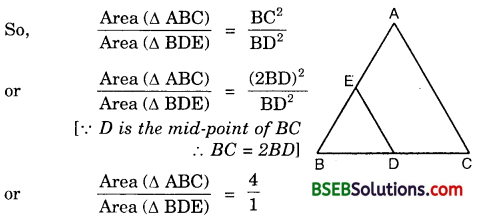∴ (C) is the correct answer.

Question 9.
Sides of two similar triangles are in the ratio 4 : 9. Areas of these triangles are in the ratio
(A) 2 : 3
(B) 4 : 9
(C) 81 : 16
(D) 16 : 81
Solution:
Since the ratio of the areas of two similar triangles is equal to the ratio of the squares of any two corresponding sides, therefore
ratio of areas = (4)² : (9)² = 16 : 81
∴ (D) is the correct answer.

## Bihar Board Class 10th Maths Chapter 6 Triangles Ex 6.4 Textbooks for Exam Preparations

Bihar Board Class 10th Maths Chapter 6 Triangles Ex 6.4 Textbook Solutions can be of great help in your Bihar Board Class 10th Maths Chapter 6 Triangles Ex 6.4 exam preparation. The BSEB STD 10th Maths Chapter 6 Triangles Ex 6.4 Textbooks study material, used with the English medium textbooks, can help you complete the entire Class 10th Maths Chapter 6 Triangles Ex 6.4 Books State Board syllabus with maximum efficiency.

## FAQs Regarding Bihar Board Class 10th Maths Chapter 6 Triangles Ex 6.4 Textbook Solutions

#### Can we get a Bihar Board Book PDF for all Classes?

Yes you can get Bihar Board Text Book PDF for all classes using the links provided in the above article.

## Important Terms

Bihar Board Class 10th Maths Chapter 6 Triangles Ex 6.4, BSEB Class 10th Maths Chapter 6 Triangles Ex 6.4 Textbooks, Bihar Board Class 10th Maths Chapter 6 Triangles Ex 6.4, Bihar Board Class 10th Maths Chapter 6 Triangles Ex 6.4 Textbook solutions, BSEB Class 10th Maths Chapter 6 Triangles Ex 6.4 Textbooks Solutions, Bihar Board STD 10th Maths Chapter 6 Triangles Ex 6.4, BSEB STD 10th Maths Chapter 6 Triangles Ex 6.4 Textbooks, Bihar Board STD 10th Maths Chapter 6 Triangles Ex 6.4, Bihar Board STD 10th Maths Chapter 6 Triangles Ex 6.4 Textbook solutions, BSEB STD 10th Maths Chapter 6 Triangles Ex 6.4 Textbooks Solutions,
Share: